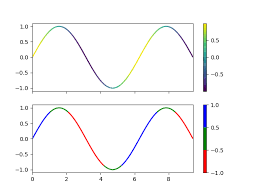# Regular oriented arrow markers

Hello,

I have a graph about 100 points.
I would like to add oriented arrows on a regular basis (1 every 25 points)
This is basically a contour plot in a “complex plan”…

Do you have any idea how I can achieve that ?

NB: Showing a rainbow curve with a colz scale would be an acceptable alternative, but I dont know if rainbow curves are supported with root…Surely something can be done. We need to know what are your data (histograms ? graphs ? … ). I you already have a small ROOT script that would be also helpful. May be also an image showing what you want to achieve.

Sure that would be great. At this time, I don’t have idea how to implement it…

I have been implementing a TGraph as following:

``````{
TGraph* gr = new TGraph("input.txt");
gr->Draw("AC");
}
``````

The input file is here :
input.txt (197.8 KB)

I can try to add marker every 25 points . but what do you mean by “oriented arrows markers” ? how should they be oriented ?

Sorry, I had prepared screenshot but forgot to put it online. Here is what I would like to achieve.
The orientation of the rotating arrows depends on the next/prev data point.

If possible, I would like to avoid marker because they are messed up when changing the X/Y scale.
Is there any chance to rotate markers in TGraph ?

ok I get it now. give me some time to write something.

### File grapharrow.C :

``````void grapharrow() {
auto gr = new TGraph("input.txt");
TExec *ex = new TExec("ex","DrawArrow();");
gr->Draw("AL");
}

void DrawArrow()
{
Int_t i,n;
Double_t x1,y1,x2,y2;
TArrow *a;
n = g->GetN();

int frequency = 25;
double dx = 0.001;

for (i=0; i<n-1; i++) {
if (i%frequency == 0) {
g->GetPoint(i,x1,y1);
g->GetPoint(i+1,x2,y2);
if (TMath::Abs(x2-x1)>dx) {
a = new TArrow(x1,y1,x2,y2,0.02,"|>");
a->SetAngle(45.);
a->Paint();
}
}
}
}

``````

can you show an example of “rainbow curve” ?

Wow that is great ! I just tested it works like a charm… May I ask you the benefit of using `gr->GetListOfFunctions()->Add(ex);` instead of passing TGraph as parameter ?

Here is a picture of what I call rainbow curveI had in mind to add a column from 0 to N and change the z-scale correspondingly to see where the curve starts and where it ends.

This adds a new painting function to the graph. This function will be executed when the graph is drawn.

One comment to this macro - one should delete created `TArrow` instances, otherwise they produce memory leak. Or simply do:

``````TArrow a(x1,y1,x2,y2,0.02,"|>");
a.SetAngle(45.);
a.Paint();
``````
2 Likes

After @linev suggestion the macro is:

``````void grapharrow() {
auto gr = new TGraph("input.txt");
TExec *ex = new TExec("ex","DrawArrow();");
gr->Draw("AL");
}

void DrawArrow()
{
Int_t i,n;
Double_t x1,y1,x2,y2;
n = g->GetN();

int frequency = 25;
double dx = 0.001;

for (i=0; i<n-1; i++) {
if (i%frequency == 0) {
g->GetPoint(i,x1,y1);
g->GetPoint(i+1,x2,y2);
if (TMath::Abs(x2-x1)>dx) {
TArrow a(x1,y1,x2,y2,0.02,"|>");
a.SetAngle(45.);
a.Paint();
}
}
}
}
``````
1 Like

Playing with canvas size, it seems that TArrow size is enlarged when X is bigger. Is there a relative size parameter to avoid such issue?

NB: In both cases arrow size is 0.02

I tried to zoom on the 1st example and I do not see any changes.
Anyway there is nothing we can do.

It seems the arrow size is driven by the width of the canvas, but independant of the height.
I though first it was related to the ratio.

``````void grapharrow() {
TCanvas *c = new TCanvas("x","", 1500,500);
auto gr = new TGraph("input.txt");
TExec *ex = new TExec("ex","DrawArrow();");
gr->Draw("AL");
}
``````
``````void grapharrow() {
TCanvas *c = new TCanvas("x","", 1500,1500);
auto gr = new TGraph("input.txt");
TExec *ex = new TExec("ex","DrawArrow();");
gr->Draw("AL");
}
``````

Both code above are rendering same arrow size, but these ones below are both smaller

``````void grapharrow() {
TCanvas *c = new TCanvas("x","", 500,1500);
auto gr = new TGraph("input.txt");
TExec *ex = new TExec("ex","DrawArrow();");
gr->Draw("AL");
}
``````

nor

``````void grapharrow() {
TCanvas *c = new TCanvas("x","", 500,500);
auto gr = new TGraph("input.txt");
TExec *ex = new TExec("ex","DrawArrow();");
gr->Draw("AL");
}
``````

My reference size is based on 500x500 canvas. I just go:

``````double scale = gPad->GetWNDC()*gPad->GetWw()/500;
a = new TArrow(x1,y1,x2,y2,0.025/scale,"|>");
``````

This really seems to be related to TArrow.
I just created a TGraph with one data point and the marker gets constant size whatever the width or height.

My guess is that TGraph is drawn based on axis, while TArrow is just a drawing that doesn’t know about axis or canvas size, but for initial positioning. Please let me know if I am wrong.

I see yes, that’s that way. Nothing we can do. You will need to change the arrow size according to the canvas width.

This topic was automatically closed 14 days after the last reply. New replies are no longer allowed.